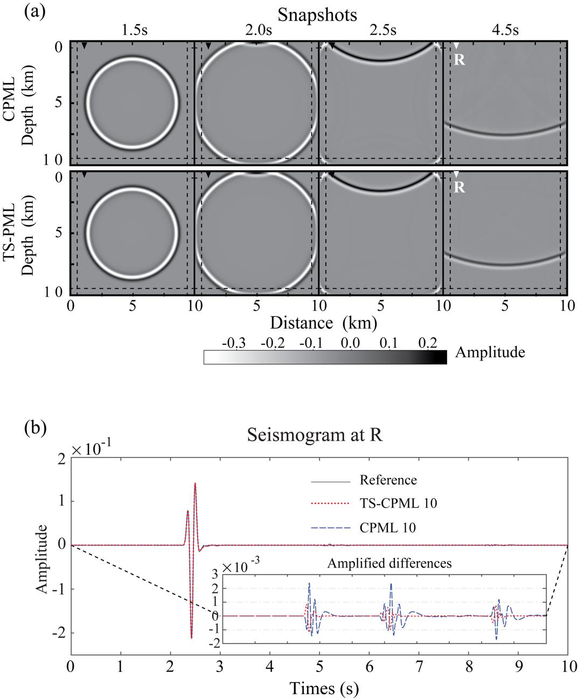News Release

# A novel implement of CPML for second-order acoustic wave equations

Peer-Reviewed Publication

Science China Pressimage: The absorbing performance of TS-CPML and the conventional CPML for the second-order acoustic wave equation. view more  Credit: ©Science China Press

Time-domain finite-difference (TDFD) method is the most dominant approach for seismic wavefield simulation. Due to the memory and computational cost of the computer, finite-difference (FD) simulations of wave equations are performed within a finite model space. As such, a proper absorbing boundary condition is required to attenuate undesired reflections from the artificial boundaries of a truncated modeling domain. Numerous approaches have been developed aiming for such an ideal absorbing boundary condition with various efficiency and stability. The perfectly matched layers (PML) method has become a widely adopted absorbing boundary method in seismic modeling and imaging for both acoustic and elastic waves in isotropic and anisotropic media. The unsplit-field CPML method is the development of the PML that can improve the absorbing performance for waves of grazing incidence and of low frequency.

The CPML method is widely used for the first-order wave equation. For a second-order equation system, there are two different ways in implementing the unsplit-field PML/CPML. The first category approach is to transform the second-order equation system to the first-order equation system, which can be solved efficiently by introducing auxiliary differential equations or by CPML method. This approach belongs to the PML implementation to the first-order differential equations. The second approach aims to transform the original wave equation into a new second-order differential equation that can be simulated with FD.

These approaches increase either the number of equations or the number of unknown parameters in the equations, which complicates the PML implementation of the second-order equation. They propose a new implementation of unsplit CPML for the second-order acoustic wave equation system without transforming the wave equation and introducing new parameters. Basically, they compute the spatial derivatives in the stretched coordinate directly by adding a correction term to the regular spatial derivative. For a second-order differential equation, one can simply apply the first-order spatial derivatives twice in the same way. Hence, they refer this two-step CPML to as TS-CPML.

The absorbing performance between the conventional CPML and the TS-CPML for the second-order acoustic wave equation is shown in Figure 1. Figure 1a shows the wavefield snapshots of the CPML (top row) and TS-CPML simulations (bottom row) at four different times, 1.5, 2.0, 2.5, and 4.5 s. Figure 1b shows the reference synthetic seismogram (accurate solution) and the CPML and TS-CPML synthetics. The three traces are almost identical, manifesting the high absorbing efficiency in both implementations. The enlarged differences indicate that TS-CPML has a better absorbing performance than the conventional CPML implementation of the second-order wave equation.

###

See the article: Fang X, Niu F. 2021. An unsplit complex frequency-shifted perfectly matched layer for second-order acoustic wave equations. Science China Earth Sciences, 64(6): 992-1004, https://doi.org/10.1007/s11430-021-9784-7

Disclaimer: AAAS and EurekAlert! are not responsible for the accuracy of news releases posted to EurekAlert! by contributing institutions or for the use of any information through the EurekAlert system.